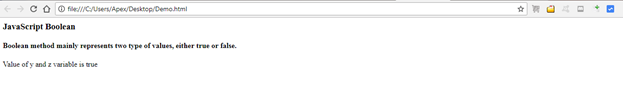Loading, please wait...## Booleans

JavaScript Booleans

In JavaScript, Boolean data type mainly represents two type of values, either true or false.

The Boolean value of 0(zero), null, undefined or the empty string is false.

For example,

``````var x = 5;
var y = 6;
var z = 5;
( x = = y)   //returns false
( x = = z)    //returns true``````

Code:

``````<html>
<body>
<h3> JavaScript Boolean </h3>
<h4> Boolean method mainly represents two type of values, either true or false.</h4>
<script>
var y = 6;
var z = 6;
document.write("Value of y and z variable is")
if (y==z)
{
document.write(" true");
}else{
document.write(" false");
}
</script>
</body>
</html> ``````

Output:JavaScript Booleans data types are useful when we need an expression which represents only two values, either True/False, On/Off, Yes/No, 0/1.